# cla

## 语法

``cla``
``cla(ax)``
``cla reset``
``cla(ax,'reset')``

## 说明

``cla` 从当前坐标区删除包含可见句柄的所有图形对象。如果对象的 `HandleVisibility` 属性设置为 `'on'`，则句柄可见。添加到坐标区的下一个绘图基于坐标区的 `ColorOrder` 和 `LineStyleOrder` 属性使用第一个颜色和线型。如果坐标区不存在，此命令会创建一个坐标区。`

``cla(ax)` 删除 `ax` 指定的坐标区、极坐标区或地理坐标区中的图形对象，而不是当前坐标区中的图形对象。`

``cla reset` 从当前坐标区删除图形对象，而不管其句柄可见性如何。它还将坐标区属性重置为默认值，但 `Position` 和 `Units` 属性除外。`

``cla(ax,'reset')` 重置指定坐标区的属性。`

## 示例

```x = linspace(0,2*pi); y1 = sin(x); plot(x,y1) hold on y2 = sin(2*x); plot(x,y2)```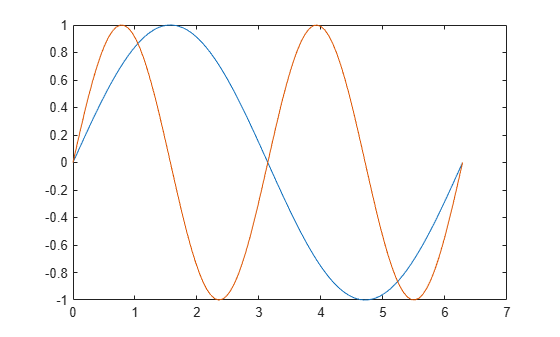`cla`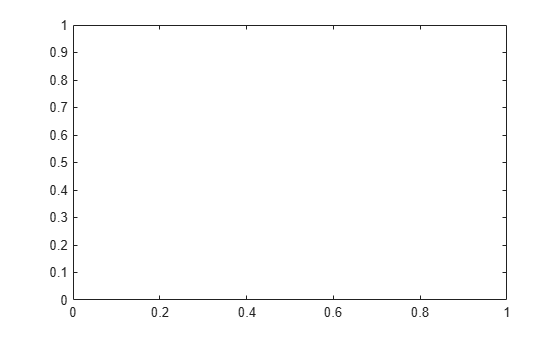`cla` 清除线图并将轴的 `ColorIndex``LineStyleIndex` 属性重置为 1。后续绘图从色序和线型序列的开头开始。例如，绘制另一个正弦波。

```y3 = sin(3*x); plot(x,y3) hold off``````tiledlayout(2,1) ax1 = nexttile; surf(ax1,peaks) ax2 = nexttile; contour(ax2,peaks)```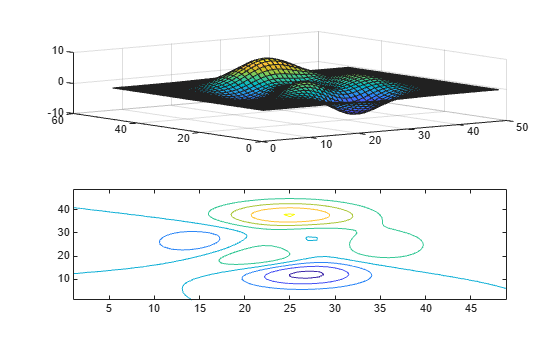`cla(ax1)`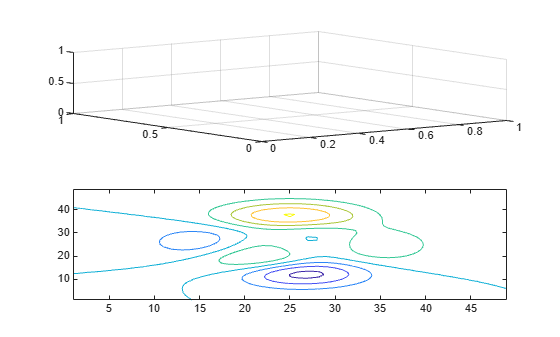`cla(ax1,'reset')````x = linspace(0,2*pi); y = sin(x); plot(x,y) axis([0 5 -2 2])```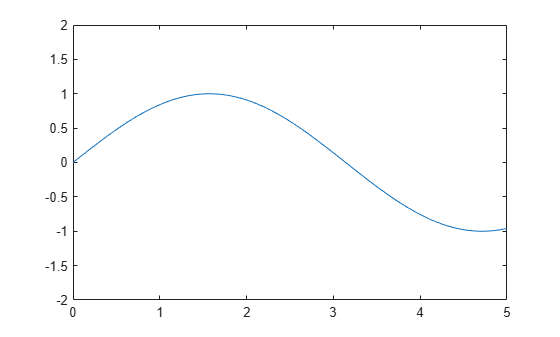`cla reset`## 算法

`cla` 命令将当前坐标区的 `ColorOrderIndex``LineStyleOrderIndex` 属性重置为 `1`